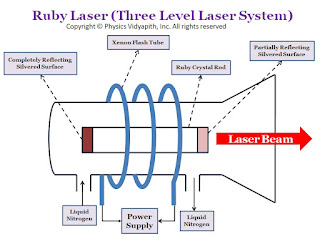## The Electric Potential at Different Points (like on the axis, equatorial, and at any other point) of the Electric Dipole

Electric Potential due to an Electric Dipole:

The electric potential due to an electric dipole can be measured at different points:

1. The electric potential on the axis of the electric dipole

2. The electric potential on the equatorial line of the electric dipole

3. The electric potential at any point of the electric dipole

1. The electric potential on the axis of the electric dipole:

Let us consider, An electric dipole AB made up of two charges of -q and +q coulomb is placed in a vacuum or air at a very small distance of $2l$. Let a point $P$ is on the axis of an electric dipole and place at a distance $r$ from the center point $O$ of the electric dipole. Now put the test charged particle $q_{0}$ at point $P$ for the measurement of electric potential due to dipole's charges.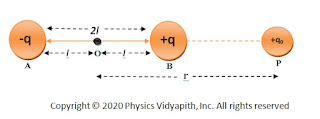The electric potential on the axis of a dipole
So Electric potential at point $P$ due $+q$ charge of electric dipole→

$V_{+q}=\frac{1}{4\pi \epsilon_{0}} \frac{q}{r-l}$

The electric potential at point $P$ due $-q$ charge of electric dipole→

$V_{-q}=-\frac{1}{4\pi \epsilon_{0}} \frac{q}{r+l}$

Electric potential is a scalar quantity. Hence the resultant potential $V$ at the point $P$ will be the algebraic sum of the potential $V_{+q}$ and $V_{-q}$. i.e. →

$V=V_{+q}+V_{-q}$

Now substitute the value of $V_{+q}$ and $V_{-q}$ in the above equation →

$V= \frac{1}{4\pi \epsilon_{0}} \frac{q}{r-l} -\frac{1}{4\pi \epsilon_{0}} \frac{q}{r+l}$

$V= \frac{1}{4\pi \epsilon_{0}} \left[ \frac{q}{r-l} - \frac{q}{r+l} \right]$

$V= \frac{q}{4\pi \epsilon_{0}} \left[ \frac{1}{r-l} - \frac{1}{r+l} \right]$

$V= \frac{q}{4\pi \epsilon_{0}} \left[ \frac{ \left( r+l \right)-\left (r-l \right)}{r^{2}-l^{2}} \right]$

$V= \frac{1}{4\pi \epsilon_{0}} \left[ \frac{2ql}{r^{2}-l^{2}} \right]$

$V= \frac{1}{4\pi \epsilon_{0}} \left[ \frac{p}{r^{2}-l^{2}} \right] \qquad \left( \because p=2ql\right)$

If $r$ is much larger then $2l$. So $l^{2}$ can be neglected in comparison to $r^{2}$. Therefore electric potential at the point $P$ due to the electric dipole is →

 $V= \frac{1}{4\pi \epsilon_{0}} \left[ \frac{p}{r^{2}} \right]$

2. The electric potential on the equatorial line of the electric dipole:

Let us consider, An electric dipole AB made up of two charges of $+q$ and $-q$ coulomb are placed in vacuum or air at a very small distance of $2l$. Let a point $P$ be on the equatorial line of an electric dipole and place it at a distance $r$ from the center point $O$ of the electric dipole. Now put the test charged particle $q_{0}$ at point $P$ for the measurement of electric potential due to dipole's charges.The electric potential on the equatorial point of a dipole
So Electric potential at point $P$ due $+q$ charge of electric dipole→

$V_{+q}=\frac{1}{4\pi \epsilon_{0}} \frac{q}{BP}$

$V_{+q}=\frac{1}{4\pi \epsilon_{0}} \frac{q}{\sqrt{r^{2}+l^{2}}}$

The electric potential at point $P$ due $-q$ charge of electric dipole→

$V_{-q}=-\frac{1}{4\pi \epsilon_{0}} \frac{q}{AP}$

$V_{-q}=-\frac{1}{4\pi \epsilon_{0}} \frac{q}{\sqrt{r^{2}+l^{2}}}$

$\therefore$ The resultant potential at point $P$ is

$V=V_{+q}+V_{-q}$

$V=\frac{1}{4\pi \epsilon_{0}} \frac{q}{\sqrt{r^{2}+l^{2}}}-\frac{1}{4\pi \epsilon_{0}} \frac{q}{\sqrt{r^{2}+l^{2}}}$

 $V=0$

Thus, the electric potential is zero on the equatorial line of a dipole (but the intensity is not zero). So No work is done in moving a charge along this line.

3. The electric potential at any point of the electric dipole:

Let us consider, an electric dipole $AB$ of length $2l$ consisting of the charge $+q$ and $-q$. Let's take a point $P$ in general and its distance is $r$ from the center point $O$ of the electric dipole AB.

Let the distance of point $P$ from the point $A$ and Point $B$ of the dipole is $PB=r_{1}$ and $PA=r_{2}$ respectively.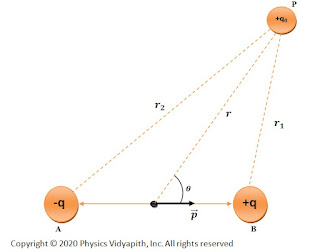The electric potential at any point of a dipole
So, The electric potential at point $P$ due to the $+q$ charge of the electric dipole is →

$V_{+q}=\frac{1}{4\pi \epsilon_{0}} \frac{q}{r_{1}}$

$V_{-q}=-\frac{1}{4\pi \epsilon_{0}} \frac{q}{r_{2}}$

The resultant potential at point $P$ is the algebraic sum of potential due to charges $+q$ and $-q$ of the dipole. That is

$V=V_{+q}+V_{-q}$

$V=\frac{1}{4\pi \epsilon_{0}} \frac{q}{r_{1}}-\frac{1}{4\pi \epsilon_{0}} \frac{q}{r_{2}}$

$V=\frac{1}{4\pi \epsilon_{0}} \left(\frac{q}{r_{1}}-\frac{q}{r_{2}} \right) \qquad(1)$
Now simplify the above equation by applying the Geometry from the figure. i.e. From the figure, Acute angle $\angle POB$, we can write as,
$r^{2}_{1}=r^{2}+l^{2}-2rlcos\theta \qquad(2)$

$r^{2}_{2}=r^{2}+l^{2}-2rlcos \left(\pi - \theta \right)$

$r^{2}_{2}=r^{2}+l^{2}+2rlcos \theta \qquad(3)$

The equation $(2)$ may be expressed as →

$r^{2}_{1}=r^{2} \left[1+ \frac{l^{2}}{r^{2}}-\frac{2l}{r}cos\theta \right]$

Taking distance $r$ much greater than the length of dipole (i.e. r>>l), so we may retain only first order term in $\frac{l}{r}$,

$\therefore r^{2}_{1}=r^{2} \left[1- \frac{2l}{r}cos\theta \right]$

$r_{1}=r \left[1- \frac{2l}{r}cos\theta \right]^{\frac{1}{2}}$

$\frac {1}{r_{1}}=\frac{1}{r} \left[1- \frac{2l}{r}cos\theta \right]^{-\frac{1}{2}}$

Now applying the binomial theorem in the above equation. So we get $\frac {1}{r_{1}}=\frac{1}{r} \left[1+ \frac{l}{r}cos\theta \right]$

Similarly,

$\frac {1}{r_{2}}=\frac{1}{r} \left[1- \frac{l}{r}cos\theta \right]$

Substituting these values in equation $(1)$, we get

$V=\frac{1}{4\pi\epsilon_{0}} \left[ \frac{q}{r} \left(1+ \frac{l}{r}cos\theta \right)-\frac{q}{r} \left(1- \frac{l}{r}cos\theta \right) \right]$

$V=\frac{1}{4\pi\epsilon_{0}}\frac{q}{r} \left[ \left(1+ \frac{l}{r}cos\theta \right)- \left(1- \frac{l}{r}cos\theta \right) \right]$

$V=\frac{1}{4\pi\epsilon_{0}}\frac{q}{r} \left[ \left(1+ \frac{l}{r}cos\theta \right)- \left(1- \frac{l}{r}cos\theta \right) \right]$

$V=\frac{1}{4\pi\epsilon_{0}}\frac{q}{r} \left[ \frac{2l cos\theta}{r}\right]$

$V=\frac{1}{4\pi\epsilon_{0}} \left[ \frac{2ql cos\theta}{r^{2}}\right]$

But $q\times 2l=p$ (dipole moment)

 $V=\frac{1}{4\pi\epsilon_{0}} \left[ \frac{p cos\theta}{r^{2}}\right]$

The vector form of the above equation can be written as →

 $V=\frac{1}{4\pi\epsilon_{0}} \left[ \frac{\overrightarrow{p} \cdot \overrightarrow{r} }{r^{2}}\right]$

The above two equations hold only under the approximation that the distance of observation point $P$ is much greater than the size of the dipole.

Special Case:
1. At axial points $\theta=0^{\circ}$,

then $cos\theta= cos 0^{\circ}=1$,

Therefore, $V=\frac{1}{4\pi\epsilon_{0}} \frac{p}{r^{2}}$
2.
3. At equatorial points $\theta=90^{\circ}$,

then $cos\theta= cos 90^{\circ}=0$,

Therefore,$\quad V=0$

Now comparing this result with the potential due to a point-charge, we see that:
1. In a fixed direction, that is , fixed $\theta$, $V\propto \frac{1}{r^{2}}$. here rather than $V \propto \frac{1}{r}$;
2.
3. Even for a fixed distance $r$, there is now a dependence on direction, that is, on $\theta$.

## Electric field intensity due to uniformly charged plane sheet and parallel sheet

Electric field intensity due to a uniformly charged infinite plane thin sheet:

Let us consider, A plane charged sheet (It is a thin sheet so it will have surface charge distribution whether it is a conducting or nonconducting sheet) whose surface charge density is $\sigma$. From symmetry, Electric field intensity is perpendicular to the plane everywhere and the field intensity must have the same magnitude on both sides of the sheet. Let point $P_{1}$ and $P_{2}$ be the two-point on the opposite side of the sheet.

To use Gaussian law, we construct a cylindrical Gaussian surface of cross-section area $\overrightarrow{dA}$, which cuts the sheet, with points $P_{1}$ and $P_{2}$. The electric field $\overrightarrow{E}$ is normal to end faces and is away from the plane. Electric field $\overrightarrow{E}$ is parallel to cross-section area $\overrightarrow{dA}$. Therefore the curved cylindrical surface does not contribute to the flux i.e. $\oint \overrightarrow{E} \cdot \overrightarrow{dA}=0$.Hence the total flux is equal to the sum of the contribution from the two end faces. Thus, we get

$\phi_{E}=\int_{A} \overrightarrow{E} \cdot \overrightarrow{dA}+\int_{A} \overrightarrow{E} \cdot \overrightarrow{dA}$

$\phi_{E}= \int_{A} E \: dA \:cos 0^{\circ} +\int_{A} E \: dA \:cos 0^{\circ} \quad \left \{\because \theta=0^{\circ} \right \}$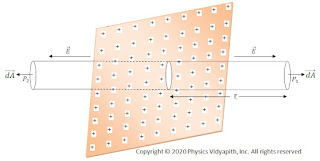Infinite plane thin sheet

$\phi_{E}= \int_{A} E \: dA +\int_{A} E \: dA$

$\phi_{E}= \int_{A} 2E \: dA$

$\phi_{E}= 2E \int_{A} \: dA$

$\phi_{E}= 2E\:A$

$\frac{q}{\epsilon_{0}}=2E\:A \qquad \left \{\because \phi_{E}=\frac{q}{\epsilon_{0}} \right \}$

$E=\frac{q}{2\epsilon_{0} A}$

$\because q=\sigma A$, So the above equation can be written as:

$E=\frac{\sigma A}{2\epsilon_{0} A}$

$E=\frac{\sigma}{2\epsilon_{0}}$

Electric field intensity due to the uniformly charged infinite conducting plane thick sheet or Plate:

Let us consider that a large positively charged plane sheet having a finite thickness is placed in the vacuum or air. Since it is a conducting plate so the charge will be distributed uniformly on the surface of the plate. Let $\sigma$ be the surface charge density of the charge

Let's take a point $P$ close to the plate at which electric field intensity has to determine. Since there is no charge inside the conducting plate, this conducting plate can be assumed as equivalent to two plane sheets of charge i.e sheet 1 and sheet 2.Plane Charged Plate

The magnitude of the electric field intensity $\overrightarrow {E_{1}}$ at point $P$ due to sheet 1 is →

$E_{1}=\frac{\sigma}{2\epsilon_{0}}$    (away from sheet 1)

The magnitude of the electric field intensity $\overrightarrow {E_{2}}$ at point $P$ due to sheet 2 is →

$E_{2}=\frac{\sigma}{2\epsilon_{0}}$    (away from sheet 2)

Since $\overrightarrow {E_{1}}$ and $\overrightarrow {E_{2}}$ are in the same direction, the magnitude of resultant intensity $\overrightarrow {E}$ at point $P$ due to both the sheet is →

$E=E_{1}+E_{2}$

$\because \quad E=\frac{\sigma}{2\epsilon_{0}}+\frac{\sigma}{2\epsilon_{0}}$

$E=\frac{\sigma}{\epsilon_{0}}$

The resultant electric field will be away from the plate. If the plate is negatively charged, the electric field intensity $\overrightarrow {E}$ would be directed toward the plate.

We have obtained the above formula for a 'plane' charged conductor. In fact, it holds for the electric field intensity 'just' outside a charged conductor of any shape.

Electric field intensity due to two Infinite Parallel Charged Sheets:

When both sheets are positively charged:

Let us consider, Two infinite, plane, sheets of positive charge, 1 and 2 are placed parallel to each other in the vacuum or air. Let $\sigma_{1}$ and $\sigma_{2}$ be the surface charge densities of charge on sheet 1 and 2 respectively.Likely positive charged sheet
Let $\overrightarrow {E_{1}}$ and $\overrightarrow {E_{2}}$ be the electric field intensities at any point due to sheet 1 and sheet 2 respectively. Then,

The electric field intensity at points $P'$ →

$E_{1}=\frac{\sigma_{1}}{2\epsilon_{0}}$    (away from sheet 1)

$E_{2}=\frac{\sigma_{2}}{2\epsilon_{0}}$    (away from sheet 2)

Since, Electric field intensities $\overrightarrow {E_{1}}$ and $\overrightarrow {E_{2}}$ are in the same direction, the magnitude of resultant intensity at point $P'$ is given by →

$E=E_{1}+E_{2}$

$E=\frac{\sigma_{1}}{2 \epsilon_{0}}+\frac{\sigma_{2}}{2 \epsilon_{0}}$

$E=\frac{1}{2 \epsilon_{0}} \left (\sigma_{1}+\sigma_{2} \right )$

If both sheets have equal charge densities $\sigma$ i.e. $\sigma_{1}=\sigma_{2}=\sigma$, Then above equation can be written as:

$E=\frac{\sigma}{ \epsilon_{0}}$

This electric field intensity would be away from both sheet 1 and sheet 2.

The electric field intensity at points $P$→

Electric field intensity at point $P$ due to sheet 1 is →

$E_{1}=\frac{\sigma_{1}}{2\epsilon_{0}}$    (away from sheet 1)

$E_{2}=\frac{\sigma_{2}}{2\epsilon_{0}}$    (away from sheet 2)

Now, both electric field intensities $\overrightarrow{E_{1}}$ and $\overrightarrow{E_{2}}$ are in opposite direction. The magnitude of resultant electric field $\overrightarrow{E}$ at point $P$ is given by

$E= E_{1}-E_{2}$

$E=\frac{\sigma_{1}}{2 \epsilon_{0}}-\frac{\sigma_{2}}{2 \epsilon_{0}}$

$E=\frac{1}{2 \epsilon_{0}} \left (\sigma_{1}-\sigma_{2} \right )$

If both sheets have equal charge densities $\sigma$ i.e. $\sigma_{1}=\sigma_{2}=\sigma$, Then above equation can be written as:

$E=0$

The electric field intensity at points $P''$ → $E_{1}=\frac{\sigma_{1}}{2\epsilon_{0}}$    (away from sheet 1)

$E_{2}=\frac{\sigma_{2}}{2\epsilon_{0}}$    (away sheet 2)

Since, Electric field intensities $\overrightarrow {E_{1}}$ and $\overrightarrow {E_{2}}$ are in the same direction, the magnitude of resultant intensity at point $P''$ is given by →

$E=E_{1}+E_{2}$

$E=\frac{\sigma_{1}}{2 \epsilon_{0}}+\frac{\sigma_{2}}{2 \epsilon_{0}}$

$E=\frac{1}{2 \epsilon_{0}} \left (\sigma_{1}+\sigma_{2} \right )$

If both sheets have equal charge densities $\sigma$ i.e. $\sigma_{1}=\sigma_{2}=\sigma$, Then above equation can be written as:

$E=\frac{\sigma}{ \epsilon_{0}}$

This electric field intensity would be away from both sheet 1 and sheet 2.

When one-sheet is positively charged and the other sheet negatively charged:

Let us consider two sheets 1 and 2 of positive and negative charge densities $\sigma_{1}$ and $\sigma_{2}$ ($\sigma_{1} > \sigma_{2}$)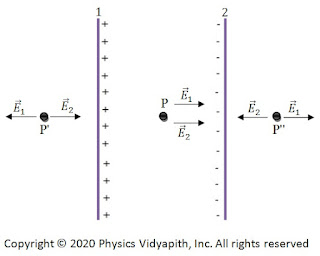Unlike charged parallel Sheet
The electric field intensities at point $P'$ →

$E_{1}=\frac{\sigma_{1}}{2\epsilon_{0}}$    (away from sheet 1)

$E_{2}=\frac{\sigma_{2}}{2\epsilon_{0}}$    (toward from sheet 2)

The magnitude of the resultant electric field $E$

$E=E_{1}-E_{2}$

$E= \frac{1}{2\epsilon_{0}} \left ( \sigma_{1}- \sigma_{2}\right )$

If both sheets have equal charge densities $\sigma$ i.e. $\sigma_{1}=\sigma_{2}=\sigma$, Then above equation can be written as:

$E=0$

The electric field intensities at point $P$ →

$E_{1}=\frac{\sigma_{1}}{2\epsilon_{0}}$    (away from sheet 1)

$E_{2}=\frac{\sigma_{2}}{2\epsilon_{0}}$    (towards sheet 2)

Since, Electric field intensities $\overrightarrow {E_{1}}$ and $\overrightarrow {E_{2}}$ are in the same direction, the magnitude of resultant intensity at point $P$ is given by →

$E=E_{1}+E_{2}$

$E=\frac{\sigma_{1}}{2 \epsilon_{0}}+\frac{\sigma_{2}}{2 \epsilon_{0}}$

$E=\frac{1}{2 \epsilon_{0}} \left (\sigma_{1}+\sigma_{2} \right )$

If both sheets have equal charge densities $\sigma$ i.e. $\sigma_{1}=\sigma_{2}=\sigma$, Then above equation can be written as:

$E=\frac{\sigma}{ \epsilon_{0}}$

The electric field intensities at point $P''$ →

$E_{1}=\frac{\sigma_{1}}{2\epsilon_{0}}$    (away from sheet 1)

$E_{2}=\frac{\sigma_{2}}{2\epsilon_{0}}$    (toward from sheet 2)

The magnitude of the resultant electric field $E$ →

$E=E_{1}-E_{2}$

$E= \frac{1}{2\epsilon_{0}} \left ( \sigma_{1}- \sigma_{2}\right )$

If both sheets have equal charge densities $\sigma$ i.e. $\sigma_{1}=\sigma_{2}=\sigma$, Then above equation can be written as:

$E=0$

From the above expression, we can conclude that the magnitude of $E$ is free from the 'position' of the point taken in the electric field between the sheet and outside the sheet. It is also shown that the electric field between the sheet is uniform everywhere and independent of separation between the sheets.

## Electric field intensity due to uniformly charged wire of infinite length

Derivation of electric field intensity due to the uniformly charged wire of infinite length: Let us consider a uniformly-charged (positively charged) wire of infinite length having a constant linear charge density (that is, a charge per unit length) $\lambda$ coulomb/meter. Let P is a point at a distance $r$ from the wire at which electric field $\overrightarrow{E}$ has to find.

Let us draw a coaxial Gaussian cylindrical surface of length $l$ through $P$. By symmetry, the magnitude $E$ of the electric field will be the same at all points on this surface and directed radially outward. Thus, for any small area taken on the surface both the electric field vector $\overrightarrow{E}$ and the area vector $\overrightarrow{dA}$ are along the same direction(radially outward). Therefore,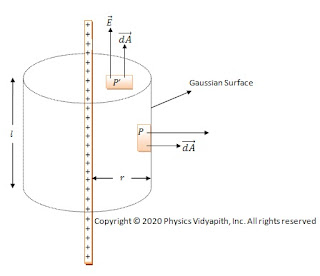Charged wire of infinite length
$d \phi_{E}= \overrightarrow{E} \cdot \overrightarrow{dA}$

$d \phi_{E}= E\: dA cos\theta$

$d \phi_{E}= E\:dA cos0^{\circ} \qquad \left(\because \theta =0^{\circ} \right)$

$d \phi_{E}= E\:dA \qquad \left(\because cos0^{\circ}=1 \right)$

Hence, the electric flux through the Gaussian surface is

$\phi_{E}= \oint{E\:dA}$

$\phi_{E}= E\:\oint{dA}$

$\phi_{E}= E\:\left(2\pi r l \right) \qquad \left\{\because \oint{dA} =2\pi r l \right\}$

The electric flux through the plane ends of the surface is zero because $\overrightarrow{E}$ and $\overrightarrow{dA}$ are at right angle everywhere on these faces ($\because \overrightarrow{E} \cdot \overrightarrow{dA}=0$).Hence, the total electric flux through the Gaussian surface is

$\phi_{E}= E\:\left(2\pi r l \right)$

But, by Gauss's law, this must be $\frac{q}{\epsilon_{0}}$, where $q$ is the net charge enclosed by the Gaussian surface. so that

$\frac{q}{\epsilon_{0}}= E\:\left(2\pi r l \right)$

$E= \frac{q}{2\pi r l \epsilon_{0}}$

for linear charge distribution → $q=\lambda l$. So the above equation can be written as

$E= \frac{\lambda l}{2\pi r l \epsilon_{0}}$

$E= \frac{\lambda}{2\pi\epsilon_{0} r }$

The vector form of the above equation can be written as:

$E= \frac{\lambda}{2\pi\epsilon_{0} r }\widehat{r}$

Where $\widehat{r}$ is unit vector in the direction of $r$. The direction of $\overrightarrow{E}$ is radially outwards(for positively charged wire).

Thus, the electric field ($E$) due to the linear charge is inversely proportional to the distance ($r$) from the linear charge and its direction is outward perpendicular to the linear charge.

Special Note: A charged cylindrical conductor behaves for external points as if the whole charge is distributed along its axis.

## Electric field intensity due to point charge by Gauss's Law

Derivation of electric field intensity due to a point charge by Gauss's Law: Let us consider, a source point charge particle of $+q$ coulomb is placed at point $O$ in space. Let's take a point $P$ on the electric field of the source point charge particle. To find the electric field intensity $\overrightarrow{E}$ at point $P$, first put the test charge particle $+q_{0}$ on the point $P$ and draw a gaussian surface which passes through the point $P$. After that take a very small area $\overrightarrow{dA}$ around the point $P$. If the distance between the source charge particle and small area $\overrightarrow {dA}$ is $r$ then electric flux passing through the small area $\overrightarrow{dA}$ →

$d\phi_{E}= \overrightarrow{E} \cdot \overrightarrow{dA}$

$d\phi_{E}= E\:dA\: cos\theta$Electric field due to point charge

from the figure, the direction between $\overrightarrow{E}$ and $\overrightarrow{dA}$ is parallel to each other i.e. the angle will be $0^{\circ}$. So the above equation can be written as →

$d\phi_{E}= E\:dA\: cos0^{\circ}$

$d\phi_{E}= E\:dA$

The electric flux passing through the entire Gaussian surface and be found by closed integration of the above equation →

$d\phi_{E}= \oint {E\:dA}$

$d\phi_{E}= E\:\oint {dA}$

$\phi_{E}= E\left(4\pi r^{2} \right) \qquad \left\{ \because \oint {dA}=4\pi r^{2} \right\}$

According to Gauss's Law → $\phi_{E}= \frac{q}{\epsilon_{0}}$ then above equation can be written as →

$\frac{q}{\epsilon_{0}}= E \left(4\pi r^{2} \right)$

$E= \frac{1}{4\pi\epsilon_{0}} \frac{q}{r^{2}}$

The above expression is the electric field intensity due to a point source charged particle.

## Normalization of the wave function of a particle in one dimension box or infinite potential well

Description of Normalization of the wave function of a particle in one dimension box or infinite potential well:

We know that the wave function for the motion of the particle along the x-axis is

$\psi_{n}(x)= A \: sin \left( \frac{n \pi x}{L} \right) \quad \left\{ Region \quad 0 \lt x \lt a \right\}$

$\psi_{n}(x)= 0 \quad \left\{ Region \quad 0 \gt x \gt a \right\}$

The total probability that the particle is somewhere in the box must be unity. Therefore,

$\int_{0}^{L} \left| \psi_{n}(x)\right|^{2}dx =1$

Now substitute the value of the wave function in the above equation. Then

$\int_{0}^{L} \left| A \: sin \left( \frac{n \pi x}{L} \right) \right|^{2}dx =1$

$\int_{0}^{L} A^{2} \: sin^{2} \left( \frac{n \pi x}{L} \right) dx =1$

$\frac{A^{2}}{2}\int_{0}^{L} \left[ 1- cos \left( \frac{2n \pi x}{L} \right) \right] dx =1$

$\frac{A^{2}}{2} \left[ x - \left( \frac{L}{2n\pi} \right) sin \left( \frac{2n \pi x}{L} \right) \right]_{0}^{L} =1$

$\frac{A^{2}}{2} \left[ L - \left( \frac{L}{2n\pi} \right) sin \left( \frac{2n \pi L}{L} \right) \right] =1$

$\frac{A^{2}}{2} \left[ L - \left( \frac{L}{2n\pi} \right) sin \left( 2n \pi \right) \right] =1$

$\frac{A^{2}}{2} \left[ L - \left( \frac{L}{2n\pi} \right) sin \left( 2n \pi \right) \right] =1$

$\frac{A^{2}}{2} \left[ L - 0 \right] =1 \qquad(\because sin2n\pi =0)$

$\frac{A^{2} L}{2} =1$

$A= \sqrt{\frac{2}{L}}$

Hence, the normalized wave function

$\psi_{n}(x)=\sqrt{\frac{2}{L}} sin \left( \frac{n \pi x}{L} \right)$

The absolute square $\left| \psi_{n}(x) \right|^{2}$ of the wave function $\psi_{n}(x)$ gives the probability density. Hence

$\left| \psi_{n}(x) \right|^{2} = \frac{2}{L} sin^{2} \left( \frac{n \pi x}{L} \right)$

The wave function for the particle in a box can be viewed in analogy with standing waves on a string. The wave function for a standing wave that has nodes at endpoints is of the form $\psi_{n}(x)= A \: sin \left( \frac{n \pi x}{L} \right)$. The condition for a standing wave can also be expressed in terms of wavelength.

$\lambda_{n}=\frac{2 \pi}{k_{n}}$

$\lambda_{n}=\frac{2 \pi}{\frac{n \pi}{L}} \qquad \left( \because k_{n}=\frac{n \pi}{L} \right)$

$\lambda_{n}=\frac{2 L}{n}$

$L= \frac{n \: \lambda_{n}}{2}$

So,

$L= \frac{\: \lambda_{1}}{2} \qquad \left( for \: n=1 \right)$

$L= \lambda_{2} \qquad \left( for \: n=2 \right)$

$L= \frac{3 \: \lambda_{3}}{2} \qquad \left( for \: n=3 \right)$

$L= 2 \lambda_{4} \qquad \left( for \: n=4 \right)$

Geo structure of wave function $\psi_{n}(x)$ and wave function's density $\left| \psi_{n}(x) \right|^{2}$.

Variation of the wave function and probability of finding the particle in a one-dimensional box:

We know that normalised wave function $\psi_{n}(x)$

$\psi_{n}(x)=\sqrt{\frac{2}{L}} sin \left( \frac{n \pi x}{L} \right)$

The probability density of wave function $\left| \psi_{n}(x) \right|$

$\left| \psi_{n}(x) \right|^{2} = \frac{2}{L} sin^{2} \left( \frac{n \pi x}{L} \right)$

Maximum Condition:

The values of $\psi_{n}(x)$ and $\left| \psi_{n}(x) \right|^{2}$ will be maximum. When

$sin \left( \frac{n \pi x}{L} \right)=1$

$sin \left( \frac{n \pi x}{L} \right )=sin \frac{\left( 2m+1 \right) \pi}{2}$

$\frac{n \pi x}{L} =\left( 2m+1 \right) \frac{ \pi}{2}$

$x =\left( 2m+1 \right) \frac{ L}{2n}$

Minima Condition:

The values of $\psi_{n}(x)$ and $\left| \psi_{n}(x) \right|^{2}$ will be minima. When

$sin \left( \frac{n \pi x}{L} \right)=0$

$sin \left( \frac{n \pi x}{L} \right)= \sin \: m\pi$

$\frac{n \pi x}{L} = \: m\pi$

$x=m\left( \frac{L}{n} \right)$

### Principle, Construction and Working of the Ruby Laser